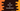# 4 different C# programs to convert decimal number to hexadecimal## C# program to convert decimal values to hexadecimal:

In this post, we will learn how to convert a decimal number to hexadecimal in C#. The decimal number system uses 10 as the base and the numerals 0, 1, 2, 3, 4, 5, 6, 7, 8, 9 are used to represent a number. Similarly, the hexadecimal system uses 16 as the base and 0, 1, 2, 3, 4, 5, 6, 7, 8, 9, a, b, c, d, e, f are used to represent a number in hexadecimal. Also, A, B, C, D, E, F can be used instead of lowercase letters.

### Algorithm to convert decimal to hexadecimal:

We can follow the below algorithm to convert a decimal number to hexadecimal:

• Divide the number by 16. Save the remainder in hexadecimal format.
• Divide the division result again by 16.
• Keep dividing the result until it become 0.
• If we combine the remainders from last to first, it will be the required hexadecimal value.

### Example to convert a decimal to hexadecimal:

Let’s try to convert 653 to hexadecimal:

• Divide 653 by 16, it is 40 and the remainder is 13. Hexadecimal value of 13 is D.
• Divide 40 by 16, it is 2 and the remainder is 8.
• Divide 2 by 16, it is 0 and the remainder is 2.

So, the hexadecimal value is 28D.

### Method 1: C# program to convert a decimal number to hexadecimal:

Let’s write down the C# program to convert a decimal value to hexadecimal:

``````using System.Text;
namespace Program
{

class Program
{
{
switch (d)
{
case 0:
return "0";
case 1:
return "1";
case 2:
return "2";
case 3:
return "3";
case 4:
return "4";
case 5:
return "5";
case 6:
return "6";
case 7:
return "7";
case 8:
return "8";
case 9:
return "9";
case 10:
return "A";
case 11:
return "B";
case 12:
return "C";
case 13:
return "D";
case 14:
return "E";
case 15:
return "F";
default:
return "";
}
}
{
StringBuilder builder = new StringBuilder();
int remainder;
while (d != 0)
{
remainder = d % 16;
d /= 16;
}

return builder.ToString();
}

static void Main(string[] args)
{
int Decimal;

Console.WriteLine("Enter the decimal number:");

}
}
}``````

Here,

• ToHexadecimalChar method is used to convert a number to hexadecimal character.
• The Main method reads the decimal number as input from the user and stores it in the integer variable Decimal. It calls ToHexadecimal method to convert it to hexadecimal and stores it in HexaDecimal variable.

If you run this program, it will print output as like below:

``````Enter the decimal number:
653

### Method 2: By using Convert.ToString method:

The Convert.ToString method is an inbuilt method of the Convert class and this method can be used to convert a decimal number to hexadecimal. This method takes two parameters to convert. The first one is the integer value to convert and the second one is the base to convert to. It will be 16 if we are converting it to hexadecimal.

Let’s use this method to convert a user given decimal value to hexadecimal:

``````namespace Program
{

class Program
{
static void Main(string[] args)
{
int Decimal;

Console.WriteLine("Enter the decimal number:");

}
}
}``````

It will print similar output:

``````Enter the decimal number:
653

### Method 3: By using Int32.ToString:

Int32.ToString method is defined as like below:

``public string ToString(string? format)``

It takes the format as the parameter and returns the converted value in string. We can pass x or X for hexadecimal conversion of an integer. x will convert it with lowercase hexadecimal characters and X will convert it with uppercase hexadecimal characters.

``````namespace Program
{

class Program
{
static void Main(string[] args)
{
int Decimal;

Console.WriteLine("Enter the decimal number:");

}
}
}``````

It will print similar output:

``````Enter the decimal number:
935

### Method 4: With String.Format method:

The String.Format method can also be used to convert an integer to hexadecimal string. It takes the format string as the first parameter and the decimal number as the second parameter and returns the hexadecimal value. We can pass {0:X} as the format string to convert a decimal to hexadecimal.

``````namespace Program
{

class Program
{
static void Main(string[] args)
{
int Decimal;

Console.WriteLine("Enter the decimal number:");